# RF Measurement Service

AET, Inc. offers you the measurement services for RF/microwave devices and circuits, taking advantage of our experience and expertise in electromagnetic fields.

• Mixed-mode differential s-parameter characterization using 4-port vector network analyzer
• S-parameter measurement using vector network analyzer ( < 50GHz)
• Creation and measurement of TEG using coplanar probe
• Measurement using spectrum analyzer (23GHz)
• Calculation of inductance and capacitance using s-parameter
• Creation of calibration modules and test fixtures based on LM/LRM methods

## Impedance Measurement Example

The Probe Station System equipped with the Air Coplanar Probe provides the impedance measurement of a microstrip line.

#### Sample

• Microstrip line, length: 30 mm
• FR-4 substrate, thickness: 0.2 mm
• Measurement frequency: 300 MHz

#### Tools used in this measurement:

• Measurement equipment: Probe Station AGPS501 (AET)
• Calibration: Calibration Kit
• Measurement probe: Air Coplanar GSG (Cascade Microtech)
• Impedance analyzer: E4991A (Keysight Technologies)
•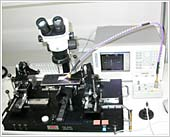Probe Station (AET)
•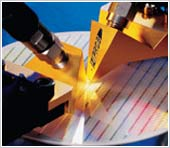## Inductance Calculation Example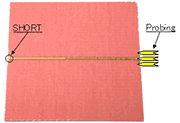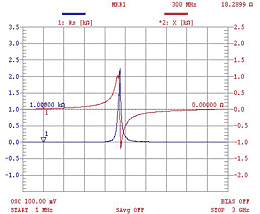( Example 1 )
Measurement example: Zo=R+jX(Ω)
At 300 MHz, Zo ＝ 0.223+j18.2899(Ω) Z(im)=jωL
Inductance L=18.2899÷(2π×300e+6) =9.71nH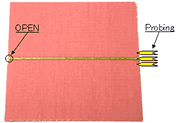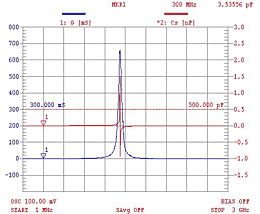( Example 2 )
Measurement example: Zo = G ＋ jX(Ω)
At 300 MHz, Zo ≒ 0.00021-j150(Ω), Z(im)=1⁄jωC
Capacitance C = 1 ÷ (150 × 2π × 300e+6) = 3.53 pF

## Applications

• Spiral inductor impedance measurement
• PCB internal capacitor thin film dielectric
• RFID Antenna
• IC package, memory stray capacitance measurement